C Program to Sort a String

In this tutorial you will learn about the C Program to Sort a String and its application with practical example.

C Program to Sort a String

In this tutorial, we will learn to create a C program that will Sort a String in C programming.

Prerequisites

Before starting with this tutorial we assume that you are best aware of the following C programming topics:

• Operators in C Programming.
• Basic Input and Output function in C Programming.
• Basic C programming.
• For loop in c programming.
• String functions of c programming.
• Conditional Statements in the C programming

Sort a String

As we all know the String is a collection of character data types. In strings, only one variable is declared which can store multiple values. First, we will give the input string to the program. Then will Sort a String by finding its length and sending it to a for loop with conditional statements in nested for loop.

With the help of this program, we can Sort a String.

Output:-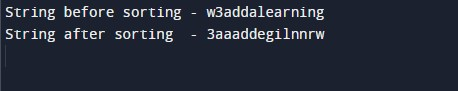In the above program, we have first initialized the required variable.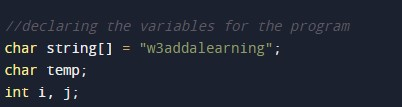• string[] = it will hold the string value.
• temp = it will hold the string value.
• i = it will hold the string value.
• j = it will hold the string value.

Finding the length of the string.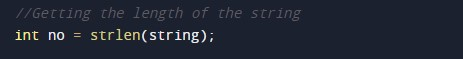Printing before sorting.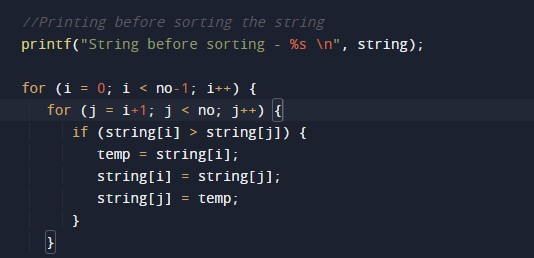Printing the output.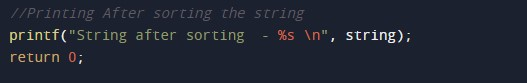In this tutorial we have learn about the C Program to Sort a String and its application with practical example. I hope you will like this tutorial.